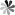Molecular Weight Calculator
Need Assistance?
+
• UK: +

# Molecular Weight Calculator

Enter the chemical formula of a compound to calculate its molar mass and elemental composition:

* Total Molecular Weight:
g/mol
Tip: Chemical formula is case sensitive. C22H30N4O c22h30n40Instructions to calculate molar mass (molecular weight) of a chemical compound:

To calculate molar mass of a chemical compound, please enter its chemical formula and click 'Calculate'.

Definitions of molecular mass, molecular weight, molar mass and molar weight:

Molecular mass (molecular weight) is the mass of one molecule of a substance and is expressed in the unified atomic mass units (u). (1 u is equal to 1/12 the mass of one atom of carbon-12)
Molar mass (molar weight) is the mass of one mole of a substance and is expressed in g/mol.

Online InquiryLoading ......